# JP5779370B2 - Electronic scanning radar apparatus, received wave direction estimation method, and program - Google Patents

## Info

Publication number
JP5779370B2
JP5779370B2 JP2011058127A JP2011058127A JP5779370B2 JP 5779370 B2 JP5779370 B2 JP 5779370B2 JP 2011058127 A JP2011058127 A JP 2011058127A JP 2011058127 A JP2011058127 A JP 2011058127A JP 5779370 B2 JP5779370 B2 JP 5779370B2
Authority
JP
Japan
Prior art keywords
frequency
spectrum
beat
based
order
Prior art date
Legal status (The legal status is an assumption and is not a legal conclusion. Google has not performed a legal analysis and makes no representation as to the accuracy of the status listed.)
Active
Application number
JP2011058127A
Other languages
Japanese (ja)
Other versions
JP2012194051A (en
Inventor

Original Assignee

Priority date (The priority date is an assumption and is not a legal conclusion. Google has not performed a legal analysis and makes no representation as to the accuracy of the date listed.)
Filing date
Publication date
Application filed by 日本電産エレシス株式会社 filed Critical 日本電産エレシス株式会社
Priority to JP2011058127A priority Critical patent/JP5779370B2/en
Publication of JP2012194051A publication Critical patent/JP2012194051A/en
Application granted granted Critical
Publication of JP5779370B2 publication Critical patent/JP5779370B2/en
Application status is Active legal-status Critical
Anticipated expiration legal-status Critical

## Images

••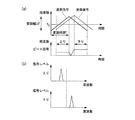•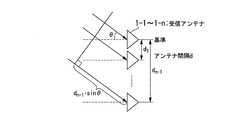•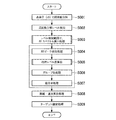••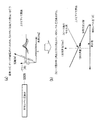•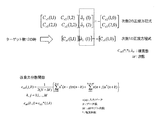•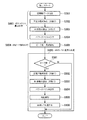•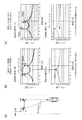•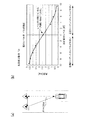•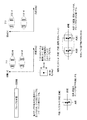•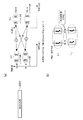•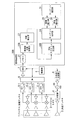•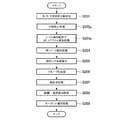•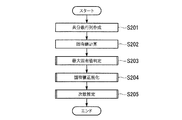•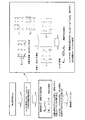•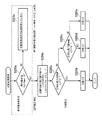•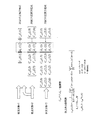•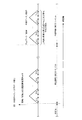•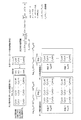## Description

The present invention relates to an electronic scanning radar apparatus suitable for in-vehicle use, a reception wave direction estimation method, and a program for detecting a target using a reflected wave from a target with respect to a radiated transmission wave.

Conventionally, in an in-vehicle electronic scanning radar apparatus, an arrival direction estimation method of an array antenna has been used as a technique for detecting the direction of an incoming wave (or a received wave) from a target (reflector).
In recent years, this arrival wave direction estimation method uses a spectrum estimation method using a high resolution algorithm such as an AR spectrum estimation method capable of obtaining a high resolution with a small number of channels (CH) and a MUSIC (MUltiple SIgnal Classification) method. (See Patent Documents 1, 2, and 3). In addition, the AR spectrum estimation method is sometimes referred to as a maximum entropy method (MEM) or a linear prediction method.
In Patent Documents 1 to 3, a technique based on a combination with DBF (Digital Beam Forming) is shown, and in some embodiments of Patent Documents 2 and 3, a technique not using DBF is also shown. Yes. For example, in the second embodiment in Patent Document 2, that is, a technique that does not use DBF, the angle detected by the high resolution algorithm for the combination of the peak of the frequency modulation in the FMCW system (upward part) and the peak of the downward part (downward part). Combination processing is performed based on the information.

JP 2006-275840 A JP 2009-156582 A JP 2009-162688 A

The AR spectrum estimation method is an algorithm with a remarkably light calculation load among high resolution algorithms. Therefore, it is possible to perform azimuth detection processing for a large number of targets existing in the distance direction (beat frequency direction in the FMCW method). Therefore, the AR spectrum estimation method is a multi-point spectrum estimation (= multi-point azimuth estimation and the like) in a case where a plurality of all detectable frequency points and a plurality of continuous specific frequency point sections are widely provided (for example, refer to Patent Document 3). It is convenient to use.
However, in a high resolution algorithm including an AR spectrum estimation method, the peak level of the detected signal is not strictly proportional to the signal strength of the incoming wave from the target.
For example, in the technique that does not use DBF, only the angle information is used without using the level information indicated by the peak of the detected signal for the combination of the peak of the frequency modulation in the FMCW system (upward part) and the peak of the downward part (downward part). (For example, refer to the second embodiment in Patent Document 2). Thus, in combination processing based only on angle information, sufficient combination accuracy cannot be ensured, and further improvement in combination accuracy is required.

The present invention has been made in view of such circumstances, and in an in-vehicle radar in which a high-resolution algorithm is applied to azimuth detection, an electronic scanning radar device that improves the combination accuracy of uplink and downlink in multipoint azimuth estimation, It is an object of the present invention to provide a received wave direction estimation method and program.

(1) The present invention is an electronic scanning radar apparatus mounted on a moving body, and includes a receiving unit configured to include a plurality of antennas that receive a received wave arriving from a target that reflects a transmitted transmission wave. A beat signal generation unit that generates a beat signal from the transmission wave and the reception wave, and a signal processing unit that detects the target based on the beat signal, and the signal processing unit preliminarily outputs the beat signal. A frequency decomposition processing unit that performs frequency decomposition into beat frequencies having a set frequency bandwidth and calculates complex number data based on the beat signal decomposed for each beat frequency, and an order based on the complex number data first azimuth spectrum which defines the secondary is calculated, a frequency point indicated as the same said beat frequency, calculating said first orientation spectrum In the frequency points corresponding to the frequency point with a second orientation spectrum is calculated that defines the order primary and the relative angles between a plurality of peaks corresponding to the first said frequency point azimuth spectrum is calculated for A target extraction unit that calculates a relative level difference between the plurality of peaks from a peak angle indicated by a peak of the second azimuth spectrum, and based on the relative level difference between the plurality of peaks, the target Is detected.

(2) Further, in the present invention according to the above invention, the signal processing unit extracts the complex data serving as a basis for calculating the first azimuth spectrum from a range of a frequency region including a plurality of beat frequencies. A frequency level detection unit is provided.

(3) Further, in the present invention according to the present invention, the frequency level detection unit is effective for spectrum estimation processing in the signal processing unit based on a size of an eigenvalue of a matrix having the complex data as an element. It is determined whether or not the data is complex number data.

(4) Moreover, this invention is provided with the azimuth | direction spectrum calculation part which calculates the said 1st azimuth spectrum based on the said complex number data in the said invention, The said target extraction part is the said calculated 1st azimuth spectrum. The second azimuth spectrum is calculated when it is determined that the plurality of peaks are present based on.

(5) Further, in the present invention according to the above aspect, the target extraction unit may perform the first step according to a correlation equation indicating a relationship between an angle difference between the plurality of peaks and a relative level difference between the plurality of peaks. The relative level difference is calculated based on a peak angle calculated based on the azimuth spectrum of 2.

(6) Moreover, the present invention is the above invention, wherein the target extraction unit is detected in each of an upstream period in which the frequency of the transmission wave is sequentially increased and a downstream period in which the frequency of the transmission wave is sequentially decreased. The peaks are grouped for each of the corresponding targets.

(7) Also, in the present invention according to the above invention, the target extraction unit performs a process of grouping the detected peaks for each target in a predetermined distance direction and angle direction with respect to the target. An area based on the distance and angle is grouped in units.

(8) Further, the present invention is characterized in that, in the above-mentioned invention, an azimuth spectrum calculation unit that calculates the first azimuth spectrum and the second azimuth spectrum according to the order estimated from the complex data is provided. .

(9) Further, in the above invention, the present invention is characterized in that the azimuth spectrum calculation unit includes an order estimation unit that estimates the order based on an eigenvalue of a matrix in which the complex number data is an element.

(10) Further, according to the present invention, in the above invention, the azimuth spectrum calculation unit performs the azimuth spectrum calculation according to the order corresponding to the complex data by an AR spectrum estimation method using an autoregressive model. It is characterized by.

(11) Further, in the present invention according to the above invention, the azimuth spectrum calculation unit performs an averaging process of a normal equation generated based on the autoregressive model, corresponding to the frequency point, and the averaging process Calculating the azimuth spectrum by the AR spectrum estimation method based on the normalized equation

(12) Further, the present invention is a reception wave direction estimation method by an electronic scanning radar apparatus mounted on a moving body, and the reception unit receives a reception wave coming from a target reflecting the transmitted transmission wave. A reception process including a plurality of antennas, a beat signal generation process in which the beat signal generation unit generates a beat signal from the transmission wave and the reception wave, and a frequency resolution processing unit is preset with the beat signal A frequency decomposition process for calculating complex number data based on the beat signal decomposed for each beat frequency by performing frequency decomposition on a beat frequency having a frequency bandwidth, and a signal processing unit based on the beat signal A signal processing process for detecting a target, wherein the frequency resolution processing unit has a preset frequency bandwidth for the beat signal. And frequency resolution in the beat frequency, the beat frequency decomposition process of calculating the complex number data based on decomposed the beat signal for each frequency, based on the complex number data, first that defines the order secondary and A second azimuth spectrum in which the order is determined to be the first order at a frequency point corresponding to the frequency point at which the first azimuth spectrum is calculated. And the target extraction unit calculates the relative angle between a plurality of peaks corresponding to the frequency point at which the first azimuth spectrum is calculated and the peak angle indicated by the peak of the second azimuth spectrum. Target extraction process for calculating the relative level difference between multiple peaks and the relative level between the multiple peaks Based on, characterized in that it comprises a the steps of detecting said target.

(13) Further, the present invention is a program for causing a computer to control the operation of estimating the direction of a received wave by an electronic scanning radar apparatus mounted on a moving body, and arrives from a target reflecting a transmitted wave transmitted. A reception process including a plurality of antennas for receiving a reception wave, a beat signal generation process in which a beat signal generation unit generates a beat signal from the transmission wave and the reception wave, and the beat signal is preset. Frequency decomposition processing for calculating complex data based on the beat signal decomposed for each beat frequency by performing frequency decomposition into beat frequencies having a frequency bandwidth, and signal processing for detecting the target based on the beat signal And the signal processing is performed to frequency the beat signal to a beat frequency having a preset frequency bandwidth. Solution to a frequency decomposition process of calculating the complex number data based on the beat signals decomposed for each of the beat frequency, on the basis of the complex data, first orientation spectrum is calculated that defines the order secondary and A second azimuth spectrum having a degree of primary is calculated at a frequency point corresponding to the frequency point indicated as the beat frequency and corresponding to the frequency point at which the first azimuth spectrum is calculated; A target for calculating a relative level difference between the plurality of peaks from a relative angle between the plurality of peaks corresponding to the frequency point at which one azimuth spectrum is calculated and a peak angle indicated by a peak of the second azimuth spectrum. Extracting the target and detecting the target based on a relative level difference between the plurality of peaks. Is a program.

As described above, according to the present invention, the reception unit is configured to include a plurality of antennas that receive a reception wave that arrives from a target that reflects a transmitted transmission wave. A beat signal generation unit generates a beat signal from the transmission wave and the reception wave. A frequency resolving unit frequency-decomposes the beat signal into beat frequencies having a preset frequency bandwidth, and calculates complex number data based on the beat signal decomposed for each beat frequency.
Based on the complex data, the first azimuth spectrum is calculated, and the first azimuth spectrum is a frequency point indicated as the same beat frequency and corresponding to the frequency point at which the first azimuth spectrum is calculated. A second azimuth spectrum set to an order lower than the order set in the process of calculating is calculated.
The target extraction unit uses the relative angle between the plurality of peaks corresponding to the frequency point at which the first azimuth spectrum is calculated, and the peak angle indicated by the peak of the second azimuth spectrum. Calculate the level difference.
The electronic scanning radar apparatus detects the target based on the relative level difference between the plurality of peaks.
As a result, by calculating the relative level difference, it is possible to add the relative level information of multi-targets of the same distance. Reliability can be improved.

1 is a block diagram showing a configuration of an electronic scanning radar apparatus according to a first embodiment of the present invention. It is a figure which shows the state into which the transmission signal and the received signal reflected by the target were input. It is a conceptual diagram explaining the received wave in a receiving antenna. It is a flowchart which shows the procedure of the process in 20 A of signal processing parts of this embodiment. It is FIG. (1) explaining each processing in signal processing part 20A. It is FIG. (2) explaining the process in 20 A of signal processing parts, respectively. It is FIG. (The 3) explaining the process in 20 A of signal processing parts, respectively. It is FIG. (The 4) explaining each in the signal processing part 20A. It is FIG. (5) explaining the process in 20 A of signal processing parts, respectively. It is FIG. (6) explaining the process in 20 A of signal processing parts, respectively. It is FIG. (7) explaining each processing in the signal processing unit 20A. It is FIG. (8) explaining the process in 20 A of signal processing parts, respectively. It is a block diagram which shows the structure of the electronic scanning radar apparatus by 2nd Embodiment of this invention. It is a flowchart which shows the procedure of the process in the signal processing part 20B of this embodiment. It is FIG. (1) explaining the process in the signal processing part 20B, respectively. It is FIG. (2) explaining the process in the signal processing part 20B, respectively. It is FIG. (The 3) explaining the process in the signal processing part 20B, respectively. It is FIG. (4) explaining the process in the signal processing part 20B, respectively. It is FIG. (5) explaining the process in the signal processing part 20B, respectively. It is FIG. (6) explaining the process in the signal processing part 20B, respectively.

<Description of AR spectrum estimation>
The AR spectrum estimation method applied to the embodiment of the present invention will be described below.
The AR spectrum estimation method is known as a spectrum estimation method for estimating a spectrum, similar to the MUSIC method, and performs an estimation process using an AR model (autoregressive model).

The AR spectrum estimation method is first modeled using an AR model indicated by a linear form, and is based on a normal equation (a matrix called an autocorrelation matrix or a covariance matrix, a vector called a right-hand side vector or a cross-correlation vector) based on input data. Also included). Furthermore, after obtaining the AR filter coefficient (AR coefficient) and the variance value of the input white noise based on the normal equation, the power spectrum is obtained and estimated using the AR coefficient and the variance value of the input white noise. . As input data, in addition to time-series data, spatial channel data such as radar of the present invention can be applied. The AR spectrum estimation method is roughly classified into a method using an autocorrelation matrix and a method using a covariance matrix, and there are an autocorrelation method (or Yulewalker method) and a Berg method as methods using an autocorrelation matrix. As a method using a covariance matrix, there are a covariance method and a modified covariance method. The improved covariance method is also referred to as a forward and backward linear prediction method (FBLP Method).

(First embodiment)
Hereinafter, an electronic scanning radar apparatus (FMCW millimeter wave radar) according to an embodiment of the present invention will be described with reference to the drawings.
FIG. 1 is a block diagram showing the configuration of the electronic scanning radar apparatus according to the embodiment.
In this figure, the electronic scanning radar apparatus 100A according to the present embodiment includes receiving antennas 1-1 to 1-n, mixers 2-1 to 2-n, a transmitting antenna 3, a distributor 4, and filters 5-1 to 5-5. n, SW (switch) 6, ADC (A / D converter) 7, control part 8, triangular wave generation part 9, VCO10, and signal processing part 20A.
The signal processing unit 20A includes a memory 21, a frequency separation processing unit 22A, a frequency resolution level detection unit 23A, a target extraction unit 24, a distance detection unit 25, a speed detection unit 26, a target determination unit 31, and an orientation spectrum calculation unit 30A. Prepare.

Next, the operation of the electronic scanning radar apparatus according to the present embodiment will be described with reference to FIG.
The receiving antennas 1-1 to 1-n receive a reflected wave, i.e., a received wave, which is transmitted from the target, with the transmitted wave reflected by the target.
The mixers 2-1 to 2-n mix the transmission waves transmitted from the transmission antenna 3 and the signals obtained by amplifying the reception waves received at the reception antennas 1-1 to 1-n by the amplifiers. A beat signal corresponding to each frequency difference is generated.
The transmission antenna 3 transmits a transmission signal obtained by frequency-modulating the triangular wave signal generated by the triangular wave generation unit 9 in a VCO (Voltage Controlled Oscillator) 10 as a transmission wave to the target.
The distributor 4 distributes the frequency-modulated transmission signal from the VCO 10 to the mixers 2-1 to 2-n and the transmission antenna 3.

Each of the filters 5-1 to 5-n performs band limitation on the beat signals of Ch1 to Chn corresponding to the reception antennas 1-1 to 1-n generated in the mixers 2-1 to 2-n, respectively. , A beat signal whose band is limited is output to SW (switch) 6.
SW6 corresponds to the sampling signal input from the control unit 8, and the beat signals of Ch1 to Chn corresponding to the receiving antennas 1-1 to 1-n that have passed through the filters 5-1 to 5-n, The signals are sequentially switched and output to an ADC (A / D converter) 7.
The ADC 7 performs A / D conversion on the beat signals of Ch1 to Chn corresponding to each of the receiving antennas 1-1 to 1-n input from the SW6 in synchronization with the sampling signal in synchronization with the sampling signal. Are converted into digital signals, and are sequentially stored in the waveform storage area of the memory 21 in the signal processing unit 20.
The control unit 8 is configured by a microcomputer or the like, and controls the entire electronic scanning radar apparatus shown in FIG. 1 based on a control program stored in a ROM (not shown) or the like.

<Principle to detect distance, relative speed, angle (azimuth)>
Next, the principle of detecting the distance, relative speed, and angle (azimuth) between the electronic scanning radar apparatus used in the signal processing unit 20A in this embodiment and the target will be briefly described with reference to the drawings.
FIG. 2 is a diagram illustrating a state in which a transmission signal and a reception signal reflected by a target are input.
The signal shown in this figure is a transmission signal obtained by frequency-modulating the signal generated in the triangular wave generation unit 9 of FIG. 1 by the VCO 10 and a reception signal received by reflecting the transmission signal by the target. In the example of this figure, the case where there is one target is shown.
As can be seen from FIG. 2A, a reception signal that is a reflected wave from the target is received with a delay in the right direction (time delay direction) in proportion to the distance from the target with respect to the signal to be transmitted. Furthermore, it varies in the vertical direction (frequency direction) with respect to the transmission signal in proportion to the relative speed with the target. Then, after the frequency conversion (Fourier transform, DTC, Hadamard transform, wave red transform, etc.) of the beat signal obtained in FIG. 2A, as shown in FIG. Each of the ascending region and the descending region has one peak value. Here, in FIG. 2A, the horizontal axis represents frequency and the vertical axis represents intensity.

The frequency resolving unit 22A performs frequency decomposition at discrete times from the sampled data of the beat signal stored in the memory 21 by frequency resolving, for example, Fourier transform, for each of the rising part (upward) and the falling part (downward) of the triangular wave. Convert. That is, the frequency resolution processing unit 22A frequency-decomposes the beat signal into beat frequencies having a preset frequency bandwidth. Then, the frequency decomposition processing unit 22A calculates complex number data based on the beat signal decomposed for each beat frequency.

As a result, as shown in FIG. 2B, a graph of the signal level for each beat frequency obtained by frequency decomposition is obtained in the rising portion and the falling portion.

Next, the distance detection unit 25 calculates the distance r by the following equation from the ascending portion target frequency fu and the descending portion target frequency fd input from the target extraction unit 24. In the formula, “•” indicates multiplication, and “/” indicates division.
r = {C · T / (2 · Δf)} · {(fu + fd) / 2}
Further, the speed detection unit 26 calculates the relative speed v from the target frequency fu of the rising portion and the target frequency fd of the falling portion input from the target extraction unit 24 by the following formula.
v = {C / (2.f0)}. {(fu-fd) / 2}
In the formula for calculating the distance r and the relative velocity v,
C: speed of light Δf: frequency modulation width of triangular wave f0 : Triangular wave center frequency T: Modulation time (rising part / falling part)
fu : Target frequency in the rising part fd : Target frequency in the descending part

Next, the receiving antennas 1-1 to 1-n in the present embodiment will be described.
FIG. 3 is a conceptual diagram illustrating the received wave at the receiving antenna.
As shown in this figure, the receiving antennas 1-1 to 1-n are arranged in an array at intervals d. The receiving antennas 1-1 to 1-n are incident waves from the target (incident waves, that is, transmitted from the transmitting antenna 3) that are incident on the axis perpendicular to the plane on which the antennas are arranged. The reflected wave from the target with respect to the transmitted wave) is input. At this time, the incoming waves are received at the same angle by the receiving antennas 1-1 to 1-n. A phase difference “dn −1 · sin θ” obtained by the same angle, for example, the angle θ and the interval d between the antennas, is generated between the adjacent receiving antennas.
By utilizing the phase difference, the angle θ can be detected by signal processing such as a high resolution algorithm for a value subjected to frequency resolution processing in the time direction for each antenna.

<Signal Processing for Received Wave in Signal Processing Unit 20A>
Next, an aspect of the signal processing unit 20A will be described with reference to FIG.
The signal processing unit 20A is configured as shown below.
The memory 21 stores time series data (ascending portion and descending portion) obtained by A / D converting the received signal in the waveform storage area by the ADC 7 in association with each antenna 1-1 to 1-n. Yes.
The frequency resolution processing unit 22A converts each beat signal corresponding to each Ch1 to Chn (each antenna 1-1 to 1-n) into a frequency component according to a preset resolution by, for example, Fourier transform. Outputs a frequency point indicating the beat frequency and complex number data of the beat frequency.
Here, the complex number data for each antenna has a phase difference depending on the angle θ, and the absolute values (reception intensity, amplitude, etc.) of each complex number data on the complex plane are equivalent.
The frequency resolving unit 22A performs frequency resolving processing such as Fourier transform (FFT) on each of the reception channel (CH) for both the rising portion (up) and the falling portion (down), and includes the beat frequency (Doppler shift). Amplitude (or power) level is calculated for each point.

The frequency resolution level detector 23 detects the amplitude level from the frequency spectrum obtained by averaging the complex number data for each reception channel. The frequency resolution level detection unit 23 exists in the vicinity of the peak indicating the maximum value (peak value) in both the rising part (upward) and the falling part (downward), and has a maximum value (quasi-peak value) smaller than the maximum value. Many frequency (distance) points are detected by setting the peripheral points including the peak to be indicated as calculation targets. The frequency resolution level detection unit 23 determines the amplitude level of the frequency spectrum obtained by averaging all the reception channels based on the set threshold value, and extracts the complex number data for each reception channel selected by the determination as valid information. The data for each reception channel at the detected point is referred to as extracted complex data. By such processing, the frequency resolution level detection unit 23 can remove information unnecessary for the subsequent orientation spectrum estimation processing. The frequency resolution level detector 23 performs the processing of the rising portion (up) and the falling portion (down) independently of each other.
The frequency resolution level detection unit 23 determines whether or not the complex number data is valid for the spectrum estimation process in the signal processing unit 20A based on the size of the eigenvalue of the matrix having the complex number data as an element. Complex data may be extracted.

The azimuth spectrum calculation unit 30A calculates an AR spectrum (= power spectrum) as the azimuth spectrum of the level detection range (that is, the extracted complex data) for both the rising portion (up) and the falling portion (down).
In addition, the azimuth spectrum calculation unit 30A calculates the dispersion value of the white noise at the same time when calculating the AR coefficient.
In the following description, the azimuth spectrum calculation unit 30A will be described as performing the AR spectrum estimation by the second-order improved covariance method (= forward-backward linear prediction method). Other spectral estimation methods can be used.

The target extraction unit 24 calculates an AR spectrum peak for each frequency point in both the ascending part (up) and the descending part (down), and performs an AR peak detection process for detecting the number of peaks and the angle for each point.
Furthermore, the target extraction unit 24 performs spectrum estimation processing by the first-order improved covariance method again for points where a plurality of peaks are detected at the same frequency point in both the rising part (up) and the falling part (down). I do.
Further, the target extraction unit 24 performs a grouping process for grouping peaks in order to facilitate the subsequent combination process. This grouping process is performed for each target. In the grouping process, each peak is targeted, and it is determined whether or not another peak falls within a certain region defined by a predetermined distance and angle with reference to a specific peak. . All groups have information about the average distance to the target and the average angle for the target for each group. Further, the information on the average relative level difference is further given to the plurality of groups (see FIG. 11).
In addition, the target extraction unit 24, as described above, groups of peaks that are grouped as detection results for upstream and downstream based on the results processed separately for the ascending part (up) and the descending part (down), respectively. Are further combined.

The distance detection unit 25 calculates the distance r to the target based on a numerical value obtained by adding the beat frequencies of the combinations of the rising region and the falling region that are sequentially input.
Further, the speed detection unit 26 calculates the relative speed v with respect to the target based on the difference between the beat frequencies of the combinations of the ascending region and the descending region that are sequentially input.

The target determination unit 31 associates the distance r, the relative speed v, and the frequency point with the target orientation calculated by the orientation spectrum calculation unit 30A.

Next, with reference to FIGS. 1 to 12, signal processing for the received wave in the signal processing unit 20A will be described.
FIG. 4 is a flowchart showing a processing procedure in the signal processing unit 20A.
The flowchart shown in FIG. 4 shows a procedure in which the first means, the second means, and the third means are combined. 5 to 12 are diagrams for explaining processing in the signal processing unit 20A shown in the flowchart of FIG.

First, in step S001, the frequency resolution processing unit 22A determines the frequency for each reception channel for each of an ascending portion (up) and a descending portion (down) of the triangular wave from the sampled data of the beat signal stored in the memory 21. Perform disassembly. Then, the frequency decomposition processing unit 22A calculates an amplitude (or power) level (frequency spectrum) from the complex number data for each point decomposed into beat frequencies.

In step S002, the frequency resolution level detector 23 detects the amplitude level based on the frequency spectrum obtained by averaging the complex number data for each reception channel. The frequency resolution level detection unit 23 exists in the vicinity of the peak indicating the maximum value (peak value) in both the rising part (upward) and the falling part (downward), and has a maximum value (quasi-peak value) smaller than the maximum value. A plurality of frequency (distance) points are detected with the surrounding points including the indicated peak as the calculation target.
Here, the process in steps S001 and S002 will be described with reference to FIG. As shown in FIG. 5 (a), the amplitude level of the beat signal with respect to the distance from the own apparatus can be graphed in each of the channels CH1 to CHn. When the target is at a specific distance point, the amplitude level of the beat signal is increased. For example, it is set as a relatively low level threshold Thf that can extract a specific continuous frequency (distance) point, and the amplitude level of the reception channel (CH) is determined according to the threshold Thf.
Complex number data for each reception channel is extracted at a distance point indicating an amplitude level larger than the threshold value Thf.

Returning to FIG. 4, in step S003, the azimuth spectrum calculation unit 30A determines the AR spectrum (= power) as the azimuth spectrum of the level detection range (that is, the extracted complex number data) for both the rising portion (up) and the falling portion (down). Spectrum).
For example, as shown in FIG. 5B, each of the AR spectra at a plurality of distance points (Pt (n−2), Pt (n−1), Pt (n), Pt (n + 1), Pt (n + 2)). The case where is calculated is shown. In the AR spectrum calculated at each distance point, each peak is concentrated in the vicinity of “−a” or “+ a”. In the AR spectrum calculated at the distance point Pt (n−2), the angle is only in the vicinity of “+ a”, and in the AR spectrum calculated at the distance point Pt (n + 2), the angle is only in the vicinity of “−a”. Suppose that In addition, this calculation result indicates the case of one of the rising part (up) and the falling part (down).

Returning to FIG. 4, in step S <b> 004, the target extraction unit 24 calculates the peak for each distance point in the ascending part (up) and the descending part (down) as the AR peak detection process, and calculates the number of peaks for each distance point. Detect the angle.
For example, in FIG. 5C, the position where the peak is detected is indicated by “black dot (•)” from the calculation result shown in FIG. In addition, at distance points (Pt (n−1), Pt (n), Pt (n + 1)) in which “black dots (•)” are surrounded by a square, points where a plurality of peaks are detected at the same distance point. Indicates. This calculation result shows the case of one of the ascending part (up) and the descending part (down).

Returning to FIG. 4, in step S005, the target extraction unit 24 performs the relative level difference calculation processing at points where a plurality of peaks are detected at the same distance point in both the rising portion (upward) and the falling portion (downward). Therefore, the spectrum estimation process by the first-order improved covariance method is performed again. A specific processing procedure will be described later.
For example, the angle difference between two peaks is obtained from the result calculated in step S005. The peak angle is obtained from the first-order AR spectrum estimation process by the method shown in FIG. The relative level difference is calculated from these relationships, that is, the relationship between the obtained two peak angles and the peak angle obtained from the first-order AR spectrum estimation processing. As the approximate expression for calculating the relative level difference, for example, a plurality of approximate polynomials may be provided in advance for each angle difference (relative angle) between two peaks, or these expressions may be tabulated.
FIG. 6B shows the relation of the peak angle by the first-order AR spectrum estimation processing with respect to the relative level difference by an approximate polynomial. When the approximate polynomial shown in this figure is a linear expression, the intercept with the vertical axis is the center angle of the angle difference between the two obtained peaks. The slope of the approximate polynomial can be determined in advance. By following such a linear format, the relative level difference between the two peaks can be calculated from the angle of the peak obtained from the first-order AR spectrum estimation process.

FIG. 7 shows a normal equation created during the process.
In the normal equation shown in FIG. 7, the normal equation when the order is second order is shown as equation (1), and the normal equation when the order is lowered is shown as equation (2).

In equations (1) and (2), the left side is the product of the correlation matrix and the AR coefficient vector, and the right side is the right side vector. Each of C xM (*, *) that is an element of a correlation matrix or an element of a right-hand side vector, and a M that is an element of an AR coefficient vector are complex numbers. M represents the order of the AR model (AR model order).
Further, C xM (*, *) that is an element of the correlation matrix or an element of the right-hand side vector is derived from an improved covariance function expressed as Expression (3).

In Expression (3), x (n) indicates input data, N indicates the number of data, M indicates the order of the AR model (AR model order), and x (n−j) or x (n + k) is appended. The letter “*” indicates a complex conjugate, and k and j indicate variables.
For example, the AR coefficient vector (AR coefficient) can be obtained by solving Expression (1) and Expression (2). From the AR coefficient, the received wave number and angle can be obtained by the following method. The first method for obtaining the received wave number and angle from the AR coefficient is a method for obtaining a power spectrum by searching for a unit circle. The second method is a method for obtaining a solution (root) of a high-order polynomial.

Here, FIG. 8 is a flowchart showing a detailed processing procedure from the above-described steps S003 to S005. The same processes as those in FIG. 4 are denoted by the same reference numerals.
The processing performed by the azimuth spectrum calculation unit 30A in step S003 can be decomposed as shown in steps S301 to S304, for example.
First, in step S301, complex number data is extracted.
In step S302, a normal equation having a second order is created based on the extracted complex number data.
In step S303, an AR coefficient and a dispersion value of white noise are calculated by solving a normal equation in which the created order is quadratic. As a calculation for solving a normal equation, a calculation method such as Cholesky decomposition can be used.
In step S304, a power spectrum is calculated from the AR coefficient calculated with the order being second order and the dispersion value of white noise.
Then, as described above, in step S004, the target extraction unit 24 detects the number of peaks and the angle based on the calculated power spectrum.

In addition, the process performed by the target extraction unit 24 in step S005 can be decomposed as shown in steps S501 to S506, for example.
First, in step S501, it is determined whether or not the number of peaks detected in step S004 is two. As a result of the determination, if the number of peaks is not 2 (step S501: NO), the relative level difference calculation process (step S005) ends. On the other hand, as a result of the determination, if the number of peaks is 2 (step S501: YES), the process proceeds to step S502.

In step S502, based on the extracted complex number data, a normal equation having a first order is created.
In step S503, a normal equation with the created order as the first order is solved to calculate the AR coefficient and the white noise variance.
In step S504, a power spectrum is calculated from the AR coefficient calculated with the order being the first order and the variance value of the white noise.
In step S505, the peak angle is detected based on the calculated power spectrum.
In step S506, based on the peak angle detected in step S505, a relative level difference calculation process described later is performed, and the relative level difference calculation process (step S005) is completed.

(Principle of relative level difference calculation process)
Next, the principle of relative level difference calculation processing will be described by taking as an example the case of estimating the orientation spectra of two targets.
FIG. 9 is a diagram showing, for two targets, a spectrum (azimuth spectrum) estimated as the second order and a spectrum (azimuth spectrum) estimated as the first order.
As shown in FIG. 9 (a), the target is located at the front front of the vehicle on which the electronic scanning radar apparatus 100A is mounted and at a position left to the left by an angle θ1 (for example, 4.8 deg (degrees)) from the front front. The case where it is arranged is shown.
FIG. 9B shows the spectrum estimation result when the reception relative level differences of the targets are equal. The angles of the two peaks Pb21 and Pb22 indicated by the spectrum estimated with the order as the second order are +4.8 degrees and 0 degrees, respectively.
The power value indicated by the vertical axis fluctuates according to the detected timing when repeated detection is performed. For this reason, FIG. 9B shows the waveform of the power detected at a certain specific timing by superimposing three data, and in the case where the results of repeated detection are further superimposed, The peak value further varies within the range indicated by the arrow. Therefore, a relative level difference cannot be obtained from the waveform shown in FIG. 9B, that is, the peak value indicated by the spectrum waveform.
The target detected under such conditions is shown in comparison with the results of spectrum estimation with the order being the first order. The peak of the spectrum estimated with the order as the first order appears at 2.4 (degrees) at a stable power level. In other words, the peak of the spectrum estimated with the order as the first order can be detected as a half angle of θ1, which is an angle for considering two targets.

On the other hand, FIG. 9C shows the spectrum estimation result when the left target has a higher reception relative level than the right target. The angles of the two peaks Pb21 and Pb22 indicated by the spectrum estimated with the order as the second order are +4.8 degrees and 0 degrees, respectively.
The power value indicated by the vertical axis fluctuates according to the detected timing when repeated detection is performed. For this reason, FIG. 9C shows the waveform of the power detected at a certain specific timing by superimposing three data, and when the results of repeated detection are further superimposed, The peak value further varies within the range indicated by the arrow. Therefore, a relative level difference cannot be obtained from the waveform shown in FIG. 9C, that is, the peak value indicated by the spectrum waveform.
However, the peak of the spectrum estimated with the order as the primary is stably detected as an angle close to the peak Pc21 detected in the secondary spectrum.
Thus, the spectrum obtained as a result of spectrum estimation with the order being the first order indicates the angle of the center of the two targets when the relative level difference is the same, but when the relative level difference occurs, the spectrum is drawn toward the higher level. This is caused by the fact that the AR spectrum estimation tries to approximate to the higher amplitude level of the received wave.

FIG. 10 is a diagram illustrating a change in peak angle due to a difference in amplitude level between two received waves.
The graph shown in FIG. 10 shows how the detected peak angle changes when the amplitude levels of the received waves from the two targets are changed, as shown in FIG.
As shown in FIG. 9A, the case where the angle θ1 having only two targets is set to a predetermined angle (for example, θ1 = 4.8 deg) will be described. In this graph, the vertical axis indicates the detected peak angle, and the horizontal axis indicates the relative level difference between the left and right. The horizontal axis indicates that the amplitude (level) of the received wave from the left target increases as it goes to the left, and the amplitude of the received wave from the right target, that is, the front target increases, as it goes to the right.
By changing the relative level difference between the left and right, the peak angle is plotted on a graph and approximated by a correlation equation (polynomial).
As shown in this graph, since the distribution is symmetrical with respect to the center angle (in this case, 2.4 deg), the correlation equation can be handled by polynomial approximation. According to the present embodiment, it is possible to calculate an accurate left / right level difference within the range of ± 10 dB from this correlation equation. Even if a level difference of ± 10 dB or more occurs, the angle approaches the angle with the larger amplitude level, so it can be determined that the level difference of the amplitude level is large.
As described above, the target extraction unit 24 calculates the spectrum (second azimuth) by reducing the order according to the correlation equation indicating the relationship between the angle difference between the plurality of peaks and the relative level difference between the plurality of peaks. The relative level difference is calculated based on the peak angle calculated based on the spectrum.

Returning to FIG. 4, in step S006, the target extraction unit 24 groups the detected peaks in units of targets in order to facilitate the combination process of the subsequent steps as the grouping process. In the grouping, groups are grouped on the basis of a predetermined distance and angle determined in advance in the distance direction and the angle direction with respect to the target with reference to each (individual) peak. Then, the group is divided into a group when there is a single group at the same distance point and a group when there are a plurality of groups. Each group has information on average distance and average angle. A plurality of groups are further provided with information on average relative level differences indicating level differences in amplitude levels between the groups (see FIG. 11).

In step S007, the target extraction unit 24 performs combination processing for combining the uplink and downlink groups that have been individually processed up to step S006.
FIG. 12A shows an example in the case where a plurality of combination patterns exist only with the angle information. Even in such a case, groups can be grouped by performing group combinations based on relative level differences.
For example, it is assumed that the conditions for determining the same group are determined as follows.
The determination condition is whether or not the angles of the respective groups match. That is, the determination condition is whether or not the angle range is included in a predetermined angle range (± α (deg)) around the reference peak. And
In addition, the determination condition is whether or not the relative level differences of the respective groups match. That is, the level in which the relative level difference of the reference peak is included in a predetermined relative level difference range (± β (dB)) Whether it is a range or not is set as a determination condition.
In FIG. 12A, the combination of the relative level +5 dB (level +5 dB) and the combination of the relative level −2 dB (level −2 dB) are determined. Note that the combination conditions are not limited to the form shown in FIG. 12, and can be used in combination with a known method such as prediction from the final cycle final result.

Returning to FIG. 4, subsequently, in step S008, the distance and relative speed in the combined group are calculated, and in step S009, the target including the known angle is determined.

As described above, the electronic scanning radar apparatus 100A can calculate the arrival direction of the received wave by the spectrum estimation method using the autoregressive model.
In the present embodiment, the frequency resolution level detector 23 functions as a first means. The orientation spectrum calculation unit 30A functions as a second means. The target extraction unit 24 functions as a third means.

(Second Embodiment)
Next, the electronic scanning radar apparatus according to the present embodiment will be described with reference to FIGS.
In the present embodiment, the order is estimated by eigenvalue calculation. For example, an eigenvalue is calculated from a covariance matrix of a cubic normal equation, and the order is estimated from the eigenvalue. Here, by determining based on the maximum (or total) eigenvalue calculated by eigenvalue calculation, a threshold for determining the amplitude level of the beat signal is provided in the same manner as the frequency resolution level detection unit 23 described above, and the continuous Make it possible to detect multiple points.
In the order estimation process, when it is estimated to be second order, first and second order AR spectrum estimation is performed.

FIG. 13 is a block diagram illustrating a configuration example of the electronic scanning radar apparatus according to the present embodiment.
Similar to the first embodiment, the signal processing unit 20B in the present embodiment performs azimuth estimation using a high resolution algorithm. The same components as those in the first embodiment shown in FIG. 1 are denoted by the same reference numerals, and differences from the first embodiment will be described below.
In this figure, the electronic scanning radar apparatus 100B according to the present embodiment includes receiving antennas 1-1 to 1-n, mixers 2-1 to 2-n, a transmitting antenna 3, a distributor 4, and filters 5-1 to 5-5. n, SW (switch) 6, ADC (A / D converter) 7, control unit 8, triangular wave generation unit 9, VCO 10, and signal processing unit 20B.
The signal processing unit 20B includes a memory 21, a frequency separation processing unit 22B, an order estimation unit 27, a target extraction unit 24, a distance detection unit 25, a speed detection unit 26, a target determination unit 31, and an orientation spectrum calculation unit 30B.

In the signal processing unit 20B, the frequency resolution processing unit 22B converts the beat signals of the ascending region and the descending region for each antenna into complex number data, and outputs the frequency point indicating the beat frequency and the complex number data to the order estimating unit 27. To do.
Next, the frequency decomposition processing unit 22B outputs the corresponding complex number data for each of the ascending region and the descending region to the azimuth spectrum calculating unit 30B. This complex data becomes a target group (a specific beat frequency continuous in the ascending region and the descending region) in each of the ascending region and the descending region.
The order estimation unit 27 performs order estimation based on the supplied complex number data. Here, the order estimation unit 27 performs maximum eigenvalue determination in the model order estimation process.
The azimuth spectrum calculation unit 30B performs a spectrum estimation process in which the order is the second order and the first order for the frequency point estimated as the second order based on the result estimated by the order estimation unit 27.
The azimuth spectrum calculation unit 30B calculates the AR spectrum (= power spectrum) of the level detection range (that is, the extracted complex number data) for both the rising part (up) and the falling part (down).
The azimuth spectrum calculation unit 30B will be described as performing the AR spectrum estimation by the improved covariance method (= forward-backward linear prediction method), but other spectrum estimation methods such as the Burg method may be used. You can also.
Further, the azimuth spectrum calculating unit 30B calculates the dispersion value of the white noise at the same time when calculating the AR coefficient.

Next, with reference to FIG. 14 to FIG. 20, signal processing for the received wave in the signal processing unit 20B will be described.
FIG. 14 is a flowchart illustrating a processing procedure in the signal processing unit 20B. Steps for performing the same processing as in FIGS. 4 and 8 are given the same reference numerals.
15 to 20 are diagrams illustrating the processing shown in FIG.

In step S002a, the order estimation unit 27 performs an order estimation process based on a calculated eigenvalue from a matrix that is a constituent part of a normal equation created based on complex number data.
FIG. 15 is a flowchart showing an implementation procedure of the order estimation processing by the order estimation unit 27.
As step S002a, in step S201, the order estimation unit 27 creates a covariance matrix (or autocorrelation matrix) that is a component of a normal equation, and performs unitary transformation.
In step S202, the order estimation unit 27 calculates eigenvalues of a unitary transformed matrix (covariance matrix or autocorrelation matrix).
FIG. 16 is a diagram illustrating eigenvalue calculation.
By performing unitary transformation, it can be converted into a real correlation matrix, and the eigenvalue calculation with the most computational load in subsequent steps can be made only with real numbers, which can greatly reduce the computation load. it can.
As shown in the present embodiment, when the order is set to the third order, the real number correlation matrix (symmetric matrix) conversion of the Hermitian matrix by the unitary transformation can be performed by an arithmetic expression shown as Expression (4) (general) For the formula, see Reference (Kikuma, “Adaptive Antenna Technology” (Ohm, 2003, pp158-160)).

In Formula (4), H attached to the right shoulder indicates Hermitian transposition.
By performing this unitary conversion, it is possible to reduce the load of calculation of eigenvalues in subsequent processing, and to expect a signal correlation suppression effect. Therefore, the eigenvalue calculation in the next step can be calculated as a complex number without performing conversion to a real correlation matrix by unitary conversion, but it is desirable to perform conversion to a real correlation matrix by unitary conversion.
Further, the eigenvalue calculation can be performed by the equations shown as Equation (5) and Equation (6).

The eigenvalue calculation may be performed by reducing the processing of the eigenvector, and an arbitrary solution algorithm can be applied in addition to directly solving the eigenequation of Equation (6). For example, iterative calculation type algorithms such as the Jacobian method, the house holder method, and the QR method can be applied.

Returning to FIG. 15, in step S203, the obtained maximum eigenvalue (maximum eigenvalue) is determined. In step S204, when the calculated eigenvalue indicates a value equal to or greater than a predetermined threshold, the order estimating unit 27 normalizes each calculated eigenvalue based on the maximum value of the calculated eigenvalue. .
In step S205, the order estimation unit 27 determines the normalized eigenvalue value based on a predetermined threshold value, and selects the order of subsequent processing based on the determination result.
A series of processing from step S203 to S205 is referred to as model order estimation processing. A specific embodiment of the model order estimation process shown in steps S203 to S205 will be described later.
Returning to FIG. 14, in step S003a, the orientation spectrum calculation unit 30B determines the order of the AR spectrum calculation process according to the estimated order, and performs the AR spectrum calculation process.

FIG. 17 is a diagram showing details of the model order estimation processing shown from step 203 to step S205 in FIG.
Step S203a is a maximum eigenvalue determination process corresponding to step S203. If the maximum eigenvalue value (λa) is smaller than the preset threshold value (λmax_th) by the determination in step S203a (step S203a: No), the reflection level is determined from the information (complex number data) obtained in step S203b. The order estimation unit 27 determines that the object is a small object, and does not perform the order estimation process and the direction detection process of the next step for the target. Since the maximum eigenvalue is equivalent (proportional to) the intensity of the input signal, the maximum eigenvalue is determined even when receiving signals due to clutter conditions unique to automotive radar such as road surface multipath. By doing, it can suppress that it becomes an incorrect azimuth | direction estimation. In addition, the order estimation unit 27 may perform determination using the total sum of the calculated eigenvalues (or the sum of the diagonal elements of the original correlation matrix) instead of the maximum eigenvalue.
That is, in step S203b, both order estimation and azimuth detection can be canceled, so that the same function as the frequency level detection (step S002) of the first embodiment can be provided.

If the maximum eigenvalue value is not smaller than the preset threshold value as a result of determination in step S203a (step S203a: Yes), the process in step S204a is a process for normalizing eigenvalues corresponding to step S204. A value obtained by dividing λx by the maximum eigenvalue λa is defined as a normalized eigenvalue λy. When the eigenvalue (signal intensity) varies depending on the distance to the target as in a radar, it can be easily determined by normalizing and relatively determining the magnitude relationship between eigenvalues.

Steps S205a to S205e are the order estimation process corresponding to step S205 performed by the order estimation unit 27.
In step S205a, the second largest eigenvalue (normalized second eigenvalue) is selected from the eigenvalues normalized in step S204a, and it is determined whether the normalized second eigenvalue is smaller than a predetermined threshold Th1. To do. As a result of the determination, when it is determined that the normalized second eigenvalue is not smaller than the predetermined threshold Th1 (step S205a: No), the process proceeds to step S205c.

In step 205b, when it is determined that the normalized second eigenvalue is smaller than the predetermined threshold Th1 as a result of the determination in step S205a (step S205a: Yes), the estimated order is determined to be primary, and estimated order information (not shown) Is stored in the storage area for storing the order, and the order estimation process is completed.

In step S205c, the third largest eigenvalue (normalized third eigenvalue) is selected from the eigenvalues normalized in step S204a, and it is determined whether the normalized third eigenvalue is smaller than a predetermined threshold Th2. To do. As a result of the determination, when it is determined that the normalized third eigenvalue is not smaller than a predetermined threshold Th2 (step S205c: No), the process proceeds to step S205e.

In step 205d, when it is determined that the normalized third eigenvalue is smaller than the predetermined threshold Th2 as a result of the determination in step S205c (step S205c: Yes), the estimated order is determined to be second and estimated order information not shown in the figure. Is stored in the storage area for storing the order, and the order estimation process is completed.

In step 205e, when it is determined that the normalized third eigenvalue is not smaller than the predetermined threshold Th2 as a result of the determination in step S205c (step S205c: No), the estimated order is determined to be third and an estimated order (not shown) The information is recorded in a storage area for storing information, and the order estimation process is completed.

As described above, in the order estimation process from step S205a to S205e, the threshold value Th1 for classifying the order 1 and the order 2 or more and the threshold value Th2 for classifying the order 2 and the order 3 are configured. By adopting such a threshold value configuration, it can be set closer to a higher-order setting that is advantageous for the AR spectrum estimation accuracy in the case of a plurality of targets, so that it can be applied to a setting specific to a vehicle-mounted radar using AR spectrum estimation.

FIG. 18 shows a normal equation created during the process.
In the normal equations shown in this figure, the normal equations when the order is from the first order to the third order are shown as Expressions (7) to (9), respectively.

In Expressions (7) to (9), the left side is the product of the correlation matrix and the AR coefficient vector, and the right side is the right side vector. Each of C xM (*, *) that is an element of a correlation matrix or an element of a right-hand side vector, and a M that is an element of an AR coefficient vector are complex numbers. M represents the order of the AR model (AR model order).
Further, C xM (*, *) that is an element of the correlation matrix or an element of the right-hand side vector is derived from an improved covariance function expressed as Expression (10).

In Expression (10), x (n) indicates input data, N indicates the number of data, M indicates the order of the AR model (AR model order), and x (n−j) or x (n + k) is attached. The letter “*” indicates a complex conjugate, and k and j indicate variables.
For example, the AR coefficient vector (AR coefficient) can be obtained by solving Expression (9) from Expression (7). From the AR coefficient, the received wave number and angle can be obtained by the following method. The first method for obtaining the received wave number and angle from the AR coefficient is a method for obtaining a power spectrum by searching for a unit circle. The second method is a method for obtaining a solution (root) of a high-order polynomial.
The orientation spectrum calculation unit 30B determines the order of the normal equation according to the estimated order. When the estimated order is 1, the azimuth spectrum calculation unit 30B obtains an AR coefficient vector based on a normal equation of order 1, and when the estimated order is 2, the AR coefficient is calculated based on a normal equation of order 1 and order 2. A vector is obtained, and when the estimated order is 3, an AR coefficient vector is obtained based on a normal equation of order 3.

Next, in the first embodiment and the second embodiment, an example that can be applied when a large number of data is acquired in the same control cycle will be described.
FIG. 19 is a time chart showing data acquisition processing in the detection cycle.
FIG. 19 shows past control (detection) cycles performed in the past from the current control (detection) cycle.
In each cycle, at least one data acquisition is performed, and one data acquisition is indicated by a triangular waveform. A triangular waveform indicates a signal modulated by the FMCW system, and detection is performed at an upward timing and at a downward timing.
Individual data acquisition is repeatedly performed with a time interval sufficient to prevent interference, and the frequency modulation period of the triangular wave does not necessarily have to be the same.
In the current control (detection) cycle, P data acquisition is performed, the first data acquisition is indicated as “current data_1 acquisition”, and the data acquisition performed in the Pth time is “current data_P acquisition”. As shown.
Based on the data acquired in the same cycle, an averaging process of various processes described later is performed.
In addition, the control (detection) cycle for which data is currently acquired is called the “current control (detection) cycle”, and the control (detection) cycle performed before the “current control (detection) cycle” is referred to as “past control (detection) cycle”. ) Cycle.

<Principle of normal equation creation process using AR model>
Next, a process for creating a normal equation using an AR model will be described in detail using an improved covariance method as an example.
A normal equation using a covariance matrix is shown in Equation (11).

In equation (11), the left side is the product of the covariance matrix C xx and the AR coefficient vector a, and the right side is the right side vector c xx .
The elements of the covariance matrix C xx are derived by the relational expression shown as Expression (12).

In the following, a specific configuration is shown as an example of performing third-order processing from 5-channel data (the maximum model order can be arbitrarily set, but in the case of 5-channel data, the third order is the maximum in the improved covariance method. (The more channels of data, the greater the maximum order that can be applied to the normal equation, and the greater the flexibility of the maximum model order that can be applied.)
The covariance matrix C xx can be expressed by a determinant of 3 rows and 3 columns, and the equation is expressed as Equation (13).

In Expression (13), each element C x3 (k, j) of the matrix represents a complex number. An arithmetic expression developed for each element is also shown. x (n), that is, (x (0), x (1), x (2), x (3), x (4)) are each complex number data, and “*” is a complex conjugate. Show.
As shown in Expression (13), the covariance matrix C xx is a Hermitian matrix (complex symmetric matrix) because of the relationship shown as Expression (14).

Similarly, a right side vector c xx in the case of performing the third order processing is shown as an expression (15).

A relational expression for deriving the variance σ v 2 of the input white noise is shown as Expression (16).

In the linear prediction by the AR model, this normal equation is derived from the condition that the least square error or the mean square error of the difference between the predicted value and the observed value (prediction error) is the minimum.
The AR coefficient is derived by solving this normal equation by a general method.
An arithmetic expression for calculating the power spectrum Sxx (ω) based on the variance σ v 2 of the input white noise calculated by Expression (16) is shown as Expression (17).

In Expression (17), ω represents an angular velocity, H AR (ω) represents a frequency characteristic from the transfer function of the AR filter at the angular velocity ω, and Svv (ω) represents a power spectrum of the input white noise at the angular velocity ω. And can be expressed as Svv (ω) = σ v 2 . Here, the angular velocity ω is converted into a phase difference of received waves when used for direction detection like the radar of the present invention.
By using the arithmetic expression shown above, it is possible to derive a spectrum having a peak characteristic that matches the target direction.

FIG. 20 is a diagram illustrating a configuration of an M-th order normal equation based on acquired complex number data and an averaging process.
The M-th order normal equation shown in FIG. 20 includes a covariance matrix that is an M-order square matrix, an AR coefficient of M rows and 1 column, and a right-side vector of M rows and 1 column as elements.
A covariance matrix and a right-hand vector are generated based on the acquired complex number data. The AR coefficient is calculated by solving a normal equation.

As shown in FIG. 19, data income is performed a plurality of times in one control (detection) cycle. A covariance matrix C xxk (t) and a right-side vector c xxk (t) are generated in the order in which the acquired complex data is acquired.
In FIG. 19, the number of times data is acquired in one control (landing) cycle is P times. The covariance matrix C xxk (t) and the right-side vector c xxk (t) are shown in order as “current time_1”,..., “ Current time_P ” corresponding to the acquired number of times.

In the present embodiment, the normal equation averaging process is performed based on data acquired within the same control (detection) cycle, and the covariance matrix C xxk (t) from “ current_1 ” to “ current_P ”. And the right side vector c xxk (t) are averaged to perform the averaging process. An equation for calculating the average covariance matrix Ave_C xxk (t) is shown in Equation (18).

In Equation (18), k1 to kP are weighting factors when performing weighted averaging.
Further, an arithmetic expression for calculating the right side vector Ave_c xxk (t) is shown in Expression (19).

In Equation (19), k1 to kP are weighting factors when performing weighted averaging.
In addition, when the eigenvalue is calculated based on the averaged normal equation, the eigenvalue of the covariance matrix of the averaged normal equation is calculated.
As described above, when a large number of data is acquired, the orientation spectrum calculation unit 30B performs an averaging process on the normal equation generated based on the autoregressive model in association with the frequency point. When the azimuth spectrum calculation unit 30B averages the normal equations, the spectrum calculated by the azimuth spectrum calculation unit 30B is stabilized, and the accuracy of the calculated spectrum can be improved.

As described above, the electronic scanning radar apparatus 100B can improve the combination accuracy of uplink and downlink of multipoint azimuth estimation without using DBF by the spectrum estimation method using the autoregressive model.
In the present embodiment, the order estimating unit 27 functions as a fourth means. The orientation spectrum calculation unit 30B functions as a second means. The target extraction unit 24 functions as a third means.

The electronic scanning radar apparatus according to the present embodiment improves detection accuracy by setting the order of a normal equation for performing spectrum estimation in the azimuth spectrum calculation unit 30B based on complex data of the detected beat frequency, and performing azimuth estimation. Can be made.

According to the first embodiment of the present invention, the vehicle-mounted radar that applies the AR spectrum estimation method to azimuth detection includes the following means, particularly when DBF is not used.
As a first means, a frequency resolution level detector 23 is provided. Here, the frequency resolution level detection unit 23 performs frequency estimation on the result calculated by the frequency resolution unit 22 (= physical point including a Doppler shift, but it is indicated as a distance point for convenience). To decide. When the frequency-resolved level detection unit 23 detects a frequency-resolved level, the frequency-resolved level detection unit 23 provides a threshold for determining the level so that continuous multipoints can be detected. The azimuth spectrum estimation in the subsequent step is performed on the frequency points in the region (multi-point region) where continuous multi-points can be detected.

As a second means, an orientation spectrum calculation unit 30A is provided. Here, AR spectrum estimation is performed in the multi-point region determined by the first means. By calculating continuous multipoint peaks, it is possible to facilitate grouping (= target unitization) of subsequent steps.

As a third means, a target extraction unit 24 is provided. Here, first, the peak of the spectrum calculated by the second means is detected, and the number of peaks and the angle are obtained. When it is determined that there are a plurality of peaks at the same distance point, AR spectrum estimation is performed by reducing the order by one, and from the peak angle of the plurality of peaks and the angle by the AR spectrum estimation result by reducing the order by one, The relative level difference of the peak is calculated by the correlation formula. The angle based on the result of AR spectrum estimation with the order lowered by one indicates the value between peaks when the set order is one lower than the number of peaks, and the value fluctuates in proportion to the relative difference in the received wave level. The characteristic to be used is used.
Next, peak detection is grouped (= target unit) for each uplink and downlink. Here, grouping is performed based on a constant distance and angle region. Finally, a combination of uplink and downlink is determined based on the target unit angle and level difference information.
By the above means, the combination of the up and down targets is determined, and the distance and relative speed can be calculated. The target is determined by the known angle, the calculated distance, and the relative speed.

Next, as a second embodiment, a fourth means can be used instead of the first means.
As a fourth means, an order estimation unit 27 is provided. Here, as preprocessing for order estimation, level thresholds are set in the same way as the first means by eigenvalue calculation of the covariance matrix and maximum (or total) eigenvalue determination by the eigenvalue calculation, and continuous multipoints are detected. I can do it.
Further, in the second means, the AR spectrum estimation as a result of the order estimation and the AR spectrum estimation with one order lowered are simultaneously performed.
In addition, when the load of eigenvalue calculation processing is heavy and cannot be calculated, instead of determining the threshold of frequency decomposition level or determining the maximum (or total) eigenvalue, the determination is made using the threshold provided with the sum of the diagonal matrix of the covariance matrix. Thus, a target frequency point may be determined.

Although the present embodiment has been described based on the configuration example used for the FMCW radar shown in FIG. 1 or FIG. 13, the present embodiment can also be applied to other antenna configurations of the FMCW scheme.
In the present embodiment, the relative level difference is calculated in the case of the order 2. However, the order 3 or more can be applied to the calculation by one lower order.
Furthermore, in the present embodiment, the improved covariance method for AR spectrum estimation has been described. However, an MFBLP method (Modified FBLP Method) using a signal subspace and a principal component AR spectrum estimation method (Principal Component AR Spectrum estimator) are used. It is also possible.

Further, the frequency resolution processing unit 22 may determine, as a frequency point for performing spectrum estimation, a result of averaging the values of adjacent frequency points based on a result calculated as a frequency point for performing spectrum estimation.
Further, based on the result calculated as the frequency point for performing spectrum estimation, the frequency resolution processing unit 22 shows a value closer to the threshold value when the value of the adjacent frequency point shows a value closer to a predetermined threshold value. It may be determined as a frequency point that performs spectrum estimation on behalf of one of the frequency points, and may be determined as a frequency point that does not perform spectrum estimation at other frequency points.

In addition, the calculation from the data acquisition to the complex number data extraction (frequency resolution processing, peak detection, etc.) should be calculated by other devices and processors (FPGA, DSP, microcomputer), etc. in addition to the calculation by the microcomputer of this embodiment. As a result, the number of data acquisitions can be increased, and the orientation estimation accuracy can be further improved.
In addition to the effects in the above applications, the relative level difference calculating means needs to recognize the peak relative level of the spectrum when the AR spectrum estimation is used for detecting the direction of the in-vehicle radar (including those other than the FMCW method). It can be widely used at appropriate places.
In the present embodiment, the power spectrum peak is calculated to obtain the number of targets and the direction. However, since it is also possible to estimate with the spectrum created without multiplying the variance value of the input white noise, the input white noise The calculation of the variance value of can be omitted. Furthermore, the direction may be estimated at the pole using a calculation for obtaining the root of a higher-order equation instead of the power spectrum.

1 and FIG. 13, a program for realizing the functions of the signal processing units 20A and 20B is recorded on a computer-readable recording medium, and the program recorded on the recording medium is read into a computer system and executed. By doing so, you may perform the signal processing which detects an azimuth | direction from a received wave. Here, the “computer system” includes an OS and hardware such as peripheral devices. The “computer system” includes a WWW system having a homepage providing environment (or display environment). The “computer-readable recording medium” refers to a storage device such as a flexible medium, a magneto-optical disk, a portable medium such as a ROM and a CD-ROM, and a hard disk incorporated in a computer system. Further, the “computer-readable recording medium” refers to a volatile memory (RAM) in a computer system that becomes a server or a client when a program is transmitted via a network such as the Internet or a communication line such as a telephone line. In addition, those holding programs for a certain period of time are also included.

The program may be transmitted from a computer system storing the program in a storage device or the like to another computer system via a transmission medium or by a transmission wave in the transmission medium. Here, the “transmission medium” for transmitting the program refers to a medium having a function of transmitting information, such as a network (communication network) such as the Internet or a communication line (communication line) such as a telephone line. The program may be for realizing a part of the functions described above. Furthermore, what can implement | achieve the function mentioned above in combination with the program already recorded on the computer system, and what is called a difference file (difference program) may be sufficient.

1-1, 1-n ... reception antenna 2-1, 2-n ... mixer 3 ... transmission antenna 4 ... distributor 5-1, 5-n ... filter 6 ... SW
8 ... Control unit 9 ... Triangle wave generation unit 10 ... VCO
20A, 20B ... Signal processing unit 21 ... Memory 22A, 22B ... Frequency resolution processing unit 23 ... Frequency resolution level detection unit 24 ... Target extraction unit 25 ... Distance detection unit 26 ... Speed detection unit 27 ... Order estimation units 30A, 30B ... Direction Spectrum calculation unit 31, 31B ... Target determination unit 100A, 100B ... Electronic scanning radar apparatus

## Claims (13)

1. An electronic scanning radar device mounted on a moving body,
A receiver configured to include a plurality of antennas for receiving a received wave arriving from a target that reflects a transmitted transmission wave;
A beat signal generation unit that generates a beat signal from the transmission wave and the reception wave;
A signal processing unit for detecting the target based on the beat signal;
With
The signal processing unit
A frequency decomposition processing unit that frequency-decomposes the beat signal into beat frequencies having a preset frequency bandwidth and calculates complex number data based on the beat signal decomposed for each beat frequency;
Based on the complex number data, a first azimuth spectrum having a second order is calculated, and is a frequency point indicated as the same beat frequency, corresponding to the frequency point at which the first azimuth spectrum is calculated. At a frequency point, a second azimuth spectrum in which the order is determined as primary is calculated, a relative angle between a plurality of peaks corresponding to the frequency point at which the first azimuth spectrum is calculated, and the second azimuth A target extraction unit that calculates a relative level difference between the plurality of peaks from a peak angle indicated by a peak of the spectrum;
With
An electronic scanning radar apparatus, wherein the target is detected based on a relative level difference between the plurality of peaks.
2. The signal processing unit
2. The electronic scanning according to claim 1, further comprising: a frequency level detection unit that extracts the complex data serving as a basis for calculating the first azimuth spectrum from a range of a frequency region including the plurality of beat frequencies. Type radar equipment.
3. The frequency level detector is
3. The method according to claim 2, wherein it is determined whether or not the complex number data is effective for spectrum estimation processing in the signal processing unit based on a size of an eigenvalue of a matrix having the complex number data as an element. The electronic scanning radar apparatus described.
4. An azimuth spectrum calculation unit for calculating the first azimuth spectrum based on the complex number data;
The target extraction unit
4. The method according to claim 1, wherein the second azimuth spectrum is calculated when it is determined that the plurality of peaks exist based on the calculated first azimuth spectrum. 5. The electronic scanning radar apparatus described.
5. The target extraction unit
The relative level difference based on the peak angle calculated based on the second azimuth spectrum according to a correlation equation indicating a relationship between an angle difference between the plurality of peaks and a relative level difference between the plurality of peaks. The electronic scanning radar apparatus according to claim 1, wherein the electronic scanning radar apparatus is calculated.
6. The target extraction unit
The peak detected in each of an upstream period in which the frequency of the transmission wave is sequentially increased and a downstream period in which the frequency of the transmission wave is sequentially decreased are grouped for each corresponding target. The electronic scanning radar apparatus according to any one of claims 1 to 5.
7. The target extraction unit
The process of grouping the detected peaks for each target is grouped in units of a region based on a predetermined distance and angle that are set in advance in a distance direction and an angle direction with respect to the target. The electronic scanning radar apparatus according to any one of claims 1 to 6.
8. The azimuth spectrum calculation part which calculates the said 1st azimuth | direction spectrum and the said 2nd azimuth | direction spectrum according to the order estimated from the said complex number data is equipped with any one of Claims 1-7 characterized by the above-mentioned. The electronic scanning radar apparatus described.
9. The orientation spectrum calculator is
The electronic scanning radar apparatus according to claim 8, further comprising: an order estimation unit configured to estimate the order based on an eigenvalue of a matrix in which the complex data is an element.
10. The orientation spectrum calculator is
10. The electron according to claim 8, wherein the calculation of the azimuth spectrum according to the order corresponding to the complex number data is performed by an AR spectrum estimation method using an autoregressive model. Scanning radar device.
11. The orientation spectrum calculator is
A normal equation generated based on the autoregressive model is averaged corresponding to the frequency point, and the azimuth spectrum is calculated by the AR spectrum estimation method based on the averaged normal equation. The electronic scanning radar apparatus according to claim 10.
12. A method of estimating a direction of a received wave by an electronic scanning radar device mounted on a moving body,
A reception process in which the reception unit includes a plurality of antennas that receive reception waves that arrive from a target that reflects the transmitted transmission waves;
A beat signal generation process in which a beat signal generation unit generates a beat signal from the transmission wave and the reception wave;
A frequency resolving process in which a frequency resolving unit frequency-decomposes the beat signal into beat frequencies having a preset frequency bandwidth and calculates complex number data based on the beat signal decomposed for each beat frequency; ,
A signal processing step in which a signal processing unit detects the target based on the beat signal;
Including
The signal processing process includes:
A frequency resolution processing step in which a frequency resolution processing unit frequency-decomposes the beat signal into beat frequencies having a preset frequency bandwidth and calculates complex number data based on the beat signal decomposed for each beat frequency When,
Based on the complex number data, a first azimuth spectrum having a second order is calculated, and is a frequency point indicated as the same beat frequency, corresponding to the frequency point at which the first azimuth spectrum is calculated. At the frequency point, a second orientation spectrum having the order determined as the first order is calculated, and the target extraction unit calculates a relative angle between a plurality of peaks corresponding to the frequency point at which the first orientation spectrum is calculated. A target extraction step of calculating a relative level difference between the plurality of peaks from a peak angle indicated by a peak of the second orientation spectrum;
Detecting the target based on a relative level difference between the plurality of peaks;
A received wave direction estimating method.
13. A program for causing a computer to control the operation of estimating the direction of a received wave by an electronic scanning radar device mounted on a moving body,
A reception process including a plurality of antennas for receiving a reception wave arriving from a target reflecting the transmitted transmission wave;
Beat signal generation processing in which a beat signal generation unit generates a beat signal from the transmission wave and the reception wave;
Frequency decomposition processing for frequency-decomposing the beat signal into beat frequencies having a preset frequency bandwidth and calculating complex data based on the beat signal decomposed for each beat frequency;
Signal processing for detecting the target based on the beat signal;
And execute
The signal processing is
Frequency decomposition processing for frequency-decomposing the beat signal into beat frequencies having a preset frequency bandwidth and calculating complex data based on the beat signal decomposed for each beat frequency;
Based on the complex number data, a first azimuth spectrum having a second order is calculated, and is a frequency point indicated as the same beat frequency, corresponding to the frequency point at which the first azimuth spectrum is calculated. At a frequency point, a second azimuth spectrum in which the order is determined as primary is calculated, a relative angle between a plurality of peaks corresponding to the frequency point at which the first azimuth spectrum is calculated, and the second azimuth A target extraction process for calculating a relative level difference between the plurality of peaks from a peak angle indicated by a peak of the spectrum;
And execute
A program for detecting the target based on a relative level difference between the plurality of peaks.
JP2011058127A 2011-03-16 2011-03-16 Electronic scanning radar apparatus, received wave direction estimation method, and program Active JP5779370B2 (en)

## Priority Applications (1)

Application Number Priority Date Filing Date Title
JP2011058127A JP5779370B2 (en) 2011-03-16 2011-03-16 Electronic scanning radar apparatus, received wave direction estimation method, and program

## Applications Claiming Priority (1)

Application Number Priority Date Filing Date Title
JP2011058127A JP5779370B2 (en) 2011-03-16 2011-03-16 Electronic scanning radar apparatus, received wave direction estimation method, and program

## Publications (2)

Publication Number Publication Date
JP2012194051A JP2012194051A (en) 2012-10-11
JP5779370B2 true JP5779370B2 (en) 2015-09-16

# Family

## Family Applications (1)

Application Number Title Priority Date Filing Date
JP2011058127A Active JP5779370B2 (en) 2011-03-16 2011-03-16 Electronic scanning radar apparatus, received wave direction estimation method, and program

## Country Status (1)

JP (1) JP5779370B2 (en)

## Families Citing this family (3)

* Cited by examiner, † Cited by third party
Publication number Priority date Publication date Assignee Title
JP2014098571A (en) * 2012-11-13 2014-05-29 Honda Elesys Co Ltd Object detection device, object detection method, object detection program, and operation control system
JP6338871B2 (en) * 2014-01-31 2018-06-06 株式会社デンソーテン Radar apparatus, vehicle control system, and signal processing method
CN105353371B (en) * 2015-12-03 2018-03-06 西安电子科技大学 Divide the sea radar target detection method of shape based on AR spectrum extensions

## Family Cites Families (5)

* Cited by examiner, † Cited by third party
Publication number Priority date Publication date Assignee Title
JPH11281729A (en) * 1998-03-31 1999-10-15 Toyota Central Res & Dev Lab Inc Beam switched radar apparatus
JP3480486B2 (en) * 1998-08-18 2003-12-22 トヨタ自動車株式会社 FM-CW radar device
JP3729127B2 (en) * 2001-12-13 2005-12-21 株式会社村田製作所 Radar
JP4910955B2 (en) * 2007-09-12 2012-04-04 株式会社デンソー Radar equipment for vehicles
JP2009162688A (en) * 2008-01-09 2009-07-23 Honda Elesys Co Ltd Electronic scanning radar device, and received wave direction estimation method and program

## Also Published As

Publication number Publication date
JP2012194051A (en) 2012-10-11

## Similar Documents

Publication Publication Date Title
US9562968B2 (en) Sensor system and method for determining target location using sparsity-based processing
US9971028B2 (en) Method and apparatus for detecting target using radar
JP6304257B2 (en) Radar apparatus, vehicle, and moving body speed detection method
CN106796282B (en) Radar system and method for determining range, relative velocity and bearing of object using continuous wave and chirp signals
US8203485B2 (en) Method of estimating direction of arrival and apparatus thereof
US20150198709A1 (en) Radar system and target detection method
DE60304692T2 (en) System and method for producing a spectrum in radar devices
JP2013015522A (en) Broad band beam formation device, broad band beam steering device and corresponding method
US7369083B2 (en) Method for performing bistatic radar functions
US9921305B2 (en) Radar apparatus and object sensing method
US8531917B2 (en) Direction of arrival estimation apparatus and method thereof

## Legal Events

Date Code Title Description
A621 Written request for application examination

Free format text: JAPANESE INTERMEDIATE CODE: A621

Effective date: 20140228

A521 Written amendment

Free format text: JAPANESE INTERMEDIATE CODE: A821

Effective date: 20140303

A977 Report on retrieval

Free format text: JAPANESE INTERMEDIATE CODE: A971007

Effective date: 20141126

A131 Notification of reasons for refusal

Free format text: JAPANESE INTERMEDIATE CODE: A131

Effective date: 20141202

A521 Written amendment

Free format text: JAPANESE INTERMEDIATE CODE: A523

Effective date: 20150112

TRDD Decision of grant or rejection written
A01 Written decision to grant a patent or to grant a registration (utility model)

Free format text: JAPANESE INTERMEDIATE CODE: A01

Effective date: 20150616

A61 First payment of annual fees (during grant procedure)

Free format text: JAPANESE INTERMEDIATE CODE: A61

Effective date: 20150713

R150 Certificate of patent or registration of utility model

Ref document number: 5779370

Country of ref document: JP

Free format text: JAPANESE INTERMEDIATE CODE: R150

R250 Receipt of annual fees

Free format text: JAPANESE INTERMEDIATE CODE: R250

R250 Receipt of annual fees

Free format text: JAPANESE INTERMEDIATE CODE: R250